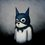# The Birthday Problem.

Recently I am taking a free course online namely stat 110 from Harvard. The course is fantastic mainly because of its instructor Joe Blitzstein and the way he teaches counter intuitive stuff in intuitive manner. Moreover the problems discussed in the lectures are phenomenal but are intricate so in order to understand them properly and as a note to myself I will write about these problems and the underlying principle they are based on.

Okay, So the First problem The Birthday problem.

Statement: There is a party and you have to find the minimum number of people that should attend the party so there is a 50% chance that at least two people will have their birthday on the same day

Solution: Say n people are attending the party so the probability that no pair of people have their birthday on the same day is given by $P(no match) = \frac{365 * 364 * \dots * (365- n + 1)}{365 ^n}$ because the first person can have his birthday on any one of the 365 days. Similarly second person can have his birthday on any day except for the day on which the first person has his birthday. So this give us 364 days and so on for the other people at the party. The multiplication symbol in between the days is obvious from the multiplication rule i.e person A can have his birthday on any of the 365 days and Person B can have his birthday on any of the 364 days, so the number of ways they both can have a birthday is $365 * 364$ and this can generalised further.

At this point we are nearly done, Probability of no match is P(no match), so the probability of match is 1 - P(no match) i.e, $P(match) = 1- P(no match)$ $P(match) = 1 - \frac{365 * 364 * \dots * (365- n + 1)}{365 ^n}$

Now we just have to substitute the various values of n and find the point at which P(match) gets bigger than 0.5.

When we substitute various values of n, it takes a minimum 23 people such that the probability of two people having birthday on the same day is 50%.

INTUITION: The result n=23 seems to be pretty counterintuitive, in the sense that the result says we require a very few people. But the important thing here is pairs of people and not the individual people themselves.

So, with 23 people how many pairs can we form $\binom{23}{2} = 253\ pairs$ now this seems pretty good because out of 253 pairs any one pair can have their birthday on the same date.Note by Gaurav Pathak
7 years, 1 month ago

This discussion board is a place to discuss our Daily Challenges and the math and science related to those challenges. Explanations are more than just a solution — they should explain the steps and thinking strategies that you used to obtain the solution. Comments should further the discussion of math and science.

When posting on Brilliant:

• Use the emojis to react to an explanation, whether you're congratulating a job well done , or just really confused .
• Ask specific questions about the challenge or the steps in somebody's explanation. Well-posed questions can add a lot to the discussion, but posting "I don't understand!" doesn't help anyone.
• Try to contribute something new to the discussion, whether it is an extension, generalization or other idea related to the challenge.

MarkdownAppears as
*italics* or _italics_ italics
**bold** or __bold__ bold
- bulleted- list
• bulleted
• list
1. numbered2. list
1. numbered
2. list
Note: you must add a full line of space before and after lists for them to show up correctly
paragraph 1paragraph 2

paragraph 1

paragraph 2

[example link](https://brilliant.org)example link
> This is a quote
This is a quote
    # I indented these lines
# 4 spaces, and now they show
# up as a code block.

print "hello world"
# I indented these lines
# 4 spaces, and now they show
# up as a code block.

print "hello world"
MathAppears as
Remember to wrap math in $$ ... $$ or $ ... $ to ensure proper formatting.
2 \times 3 $2 \times 3$
2^{34} $2^{34}$
a_{i-1} $a_{i-1}$
\frac{2}{3} $\frac{2}{3}$
\sqrt{2} $\sqrt{2}$
\sum_{i=1}^3 $\sum_{i=1}^3$
\sin \theta $\sin \theta$
\boxed{123} $\boxed{123}$